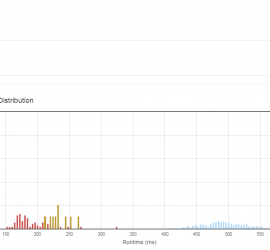# Leetcode

## [Leetcode] Sum Root to Leaf Numbers

Given a binary tree containing digits from 0-9 only, each root-to-leaf path could represent a number. An example is the root-to-leaf path 1->2->3 which represents the number 123. Find the total sum of all root-to-leaf numbers. For example, 1 / \ 2 3 The root-to-leaf path 1->2 represents the number […]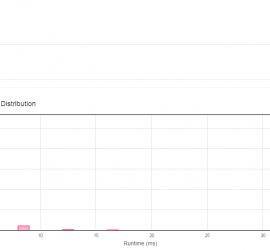## [Leetcode] Longest Consecutive Sequence

Given an unsorted array of integers, find the length of the longest consecutive elements sequence. For example, Given [100, 4, 200, 1, 3, 2], The longest consecutive elements sequence is [1, 2, 3, 4]. Return its length: 4. Your algorithm should run in O(n) complexity. 10/10/2015 udpate use an unordered_set […]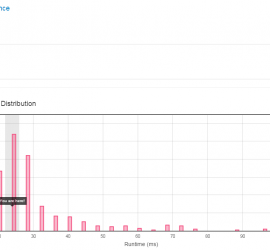Given two words (start and end), and a dictionary, find all shortest transformation sequence(s) from start to end, such that: Only one letter can be changed at a time Each intermediate word must exist in the dictionary For example, Given: start = “hit” end = “cog” dict = [“hot”,”dot”,”dog”,”lot”,”log”] Return […]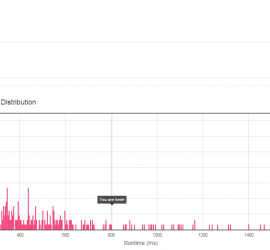Given two words (beginWord and endWord), and a dictionary, find the length of shortest transformation sequence from beginWord to endWord, such that: Only one letter can be changed at a time Each intermediate word must exist in the dictionary For example, Given: start = “hit” end = “cog” dict = […]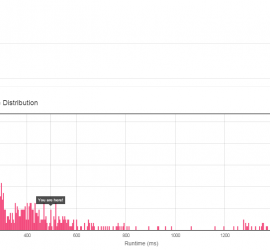## [leetcode] Populating Next Right Pointers in Each Node II

Follow up for problem “Populating Next Right Pointers in Each Node“. What if the given tree could be any binary tree? Would your previous solution still work? Note: You may only use constant extra space. For example, Given the following binary tree, 1 / \ 2 3 / \ \ […]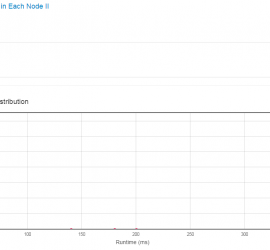## [leetcode] Populating Next Right Pointers in Each Node

Given a binary tree struct TreeLinkNode { TreeLinkNode *left; TreeLinkNode *right; TreeLinkNode *next; } Populate each next pointer to point to its next right node. If there is no next right node, the next pointer should be set to NULL. Initially, all next pointers are set to NULL. Note: You […]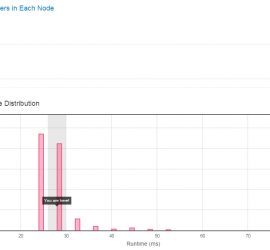## [leetcode] Distinct Subsequences3

Given a string S and a string T, count the number of distinct subsequences of T in S. A subsequence of a string is a new string which is formed from the original string by deleting some (can be none) of the characters without disturbing the relative positions of the […]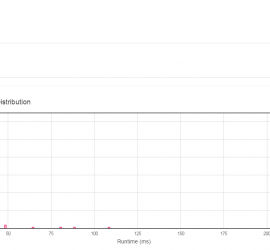## [leetcode] Binary Tree Level Order Traversal II

Given a binary tree, return the bottom-up level order traversal of its nodes’ values. (ie, from left to right, level by level from leaf to root). For example: Given binary tree {3,9,20,#,#,15,7}, 3 / \ 9 20 / \ 15 7 return its bottom-up level order traversal as: [ [15,7], […]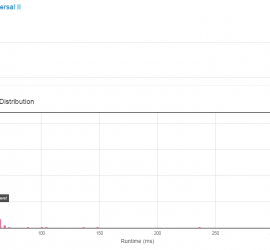## [leetcode] Construct Binary Tree from Inorder and Postorder Traversal

Given inorder and postorder traversal of a tree, construct the binary tree. Note: You may assume that duplicates do not exist in the tree. // in inorder traversal sequence, the nodes are organized as [left] [root] [right] // in postorder traversal sequence, the nodes are organized as [left] [right] [root] […]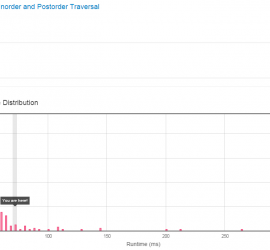## [leetcode] RecoverBinary Search Tree

Two elements of a binary search tree (BST) are swapped by mistake. Recover the tree without changing its structure. Note: A solution using O(n) space is pretty straight forward. Could you devise a constant space solution? confused what “{1,#,2,3}” means? > read more on how binary tree is serialized on […]